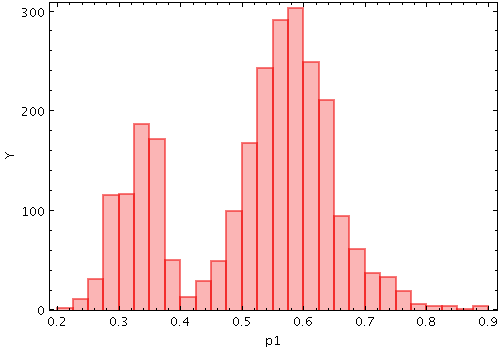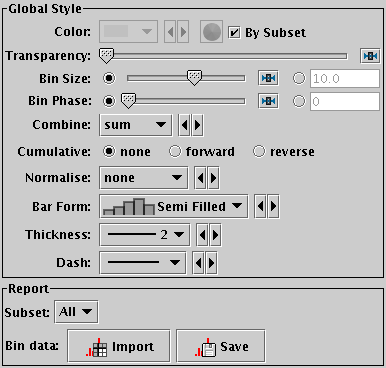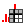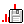Next Previous Up Contents
Next: KDE Form
Up: Plot Forms
Previous: Fill Form

#### A.4.5.21 Histogram FormExample Histogram plotHistogram form configuration panel

The Histogram form () plots a histogram generated by binning samples along the X axis.

This form may be used in the Histogram, Plane or Time plot windows.

These options always appear in the form configuration panel:

Colour
Selects the basic colour for the dataset.
Transparency
Adjusts the transparency of the bars or line. For bar styles which are already partially transparent, this fades them further.
Combine
Defines how values contributing to the same bin are combined together to produce the value assigned to that bin, and hence its height. The combined values are those given by the Weight coordinate, but if no weight is supplied, a weighting of unity is assumed.

The available options are:

• `sum`: the sum of all the combined values per bin
• `sum-per-unit`: the sum of all the combined values per unit of bin size
• `count`: the number of non-blank values per bin (weight is ignored)
• `count-per-unit`: the number of non-blank values per unit of bin size (weight is ignored)
• `mean`: the mean of the combined values
• `median`: the median of the combined values (may be slow)
• `q1`: the first quartile of the combined values (may be slow)
• `q3`: the third quartile of the combined values (may be slow)
• `min`: the minimum of all the combined values
• `max`: the maximum of all the combined values
• `stdev`: the sample standard deviation of the combined values
• `hit`: 1 if any values present, NaN otherwise (weight is ignored)

Bar Form
Type of histogram bars: filled, open, steps, spikes etc.
Thickness
Controls line thickness where applicable. This is only relevant for bar styles that draw a finite thickness line, so has no effect for solid bars.
Dash
Controls the dash pattern of the line (solid, dots, dashes etc). This is only relevant for bar styles that draw a finite thickness line, so has no effect for solid bars.
And these options appear in the form configuration panel for the Plane window, or the Bins control () for the Histogram window:
Bin Size
A scale for the width of bins that are shown on the screen. There are two ways to specify this. If the left-hand radio button is selected, the adjacent slider will adjust the bin size, which is also affected by the actual width of the plotting window in pixels. Slide the slider left to get narrower bins or right to get wider ones. If the right-hand radio button is selected, you can enter a numeric value giving the actual width in data units of each bar (for a logarithmic X axis this value is a factor).
Bin Phase
Controls where the horizontal zero point for binning is set. For instance if your bin size is 1, it controls whether bin boundaries are at 0, 1, 2, .. or 0.5, 1.5, 2.5, ... etc. If the slider is at either end of the scale, there will be a bin boundary at X=0 (linear X axis) or X=1 (logarithmic X axis).
Cumulative
If set to forward or reverse, the histogram bars are plotted cumulatively; each bin includes the counts from all previous bins in the direction of negative or positive infinity.
Normalise
Defines how, if at all, the bars are normalised. The available options are:
• none: No normalisation is performed.
• area: The total area of histogram bars is normalised to unity. For cumulative plots, this behaves like height.
• unit: Histogram bars are scaled by the inverse of the bin width in data units. For cumulative plots, this behaves like none.
• maximum: The height of the tallest histogram bar is normalised to unity. For cumulative plots, this behaves like height.
• height: The total height of histogram bars is normalised to unity.
When used in the Time plot only, additional options per_second, per_day etc are available corresponding to the frequency over the named time unit.

The Report panel gives you access to the bin values calculated by the plot:

Bin Data
This allows you to export the bin values that you can see in the plot in the form of a table. The table has a row for each bin in the plotted histogram, with columns giving the mid-point, lower bound and upper bound of each bin on the X axis, and its height. The Import () option loads the data as a new table in the TOPCAT application, and the Save () option lets you save it directly to disk in one of the available table formats. This information is also available from the Export menu.

Next Previous Up Contents
Next: KDE Form
Up: Plot Forms
Previous: Fill Form

TOPCAT - Tool for OPerations on Catalogues And Tables